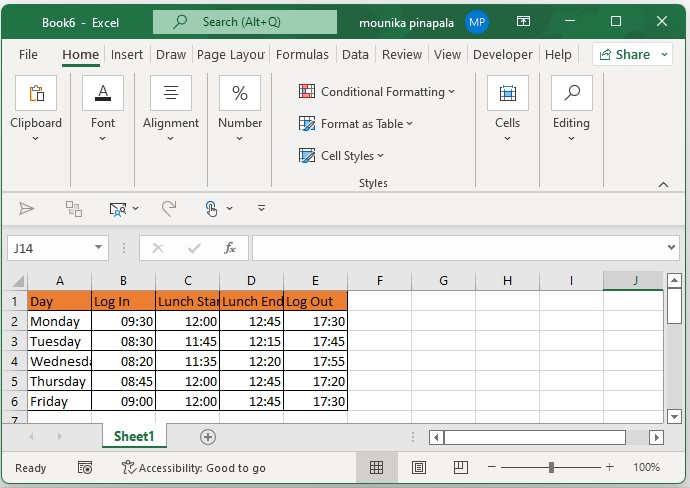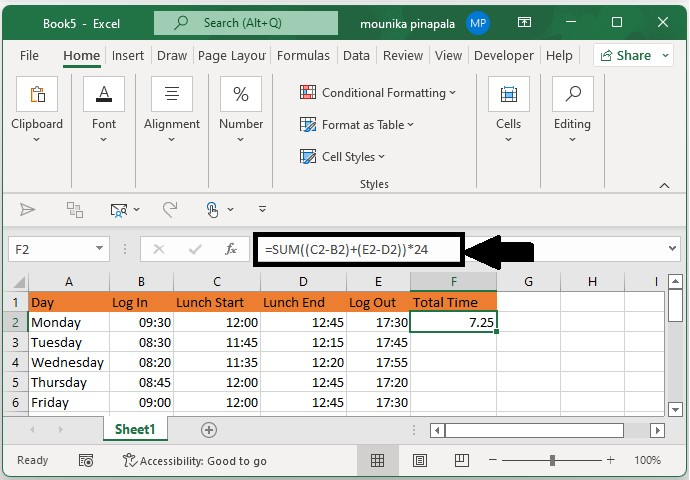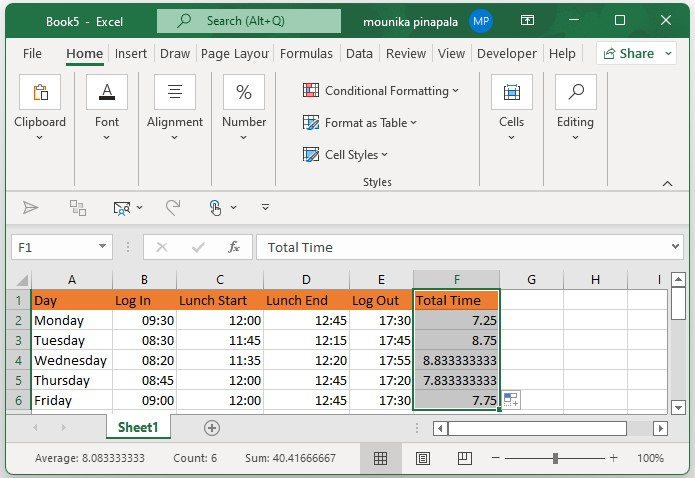# How to calculate hours worked and minus lunch time in Excel?

Whenever you work for a certain organization, you need to log in and log out at the starting and end of every workday, and counting the total working hours, minus the lunch time of a day will make it quick for you to calculate the salary based on the amount of time you have worked.

I have the following daily attendance record, which has the log-in time, the log-out time, the start time, and the end time of lunch each day in a worksheet. Now, I want to calculate the total hours worked each day with the lunch time minus.

## Step 1

Open an Excel sheet and enter the data which includes the day, log in, log out, lunch start, and lunch end timings in it as shown in the below screenshot for your reference.## Step 2

Now you need to enter the below given formula in the cell F2 and press enter key it will calculates the total time as shown in the below screenshot for your reference.

=SUM((C2-B2)+(E2-D2))*24Note − In the above given formula, C2 is the start time of the lunch, B2 is the time of the log in, E2 is the time logged out, and D2 is the end time of the lunch. You can also change this as per your wish.

## Step 3

Now you need to drag the fill handle down to the cells that you want to apply the formula, and now you will see that all the total hours excluding lunchtime are calculated at once as shown in the below screenshot for your reference.Tip − Consider if there are fixed log in and logout times and fixed lunch time such as 1 hour, then you can just enter the below formula to calculate the worked hours a day.

=D2-B2-C2

## Conclusion

In this tutorial, we used a simple example to demonstrate how you can calculate the total number of working hours minus the lunch time in Excel.

Updated on: 03-Feb-2023

704 Views